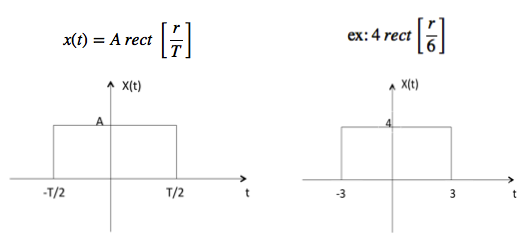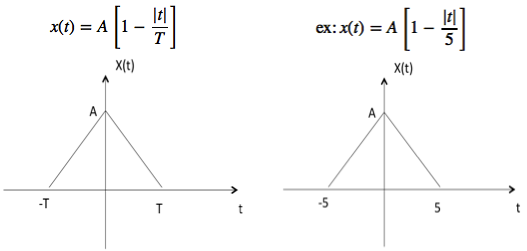# Signals Basic Types

Here are a few basic signals:

### Unit Step Function

Unit step function is denoted by u(t). It is defined as u(t) = $\left\{\begin{matrix}1 & t \geqslant 0\\ 0 & t<0 \end{matrix}\right.$• It is used as best test signal.
• Area under unit step function is unity.

### Unit Impulse Function

Impulse function is denoted by δ(t). and it is defined as δ(t) = $\left\{\begin{matrix}1 & t = 0\\ 0 & t\neq 0 \end{matrix}\right.$$$\int_{-\infty}^{\infty} δ(t)dt=u (t)$$

$$\delta(t) = {du(t) \over dt }$$

### Ramp Signal

Ramp signal is denoted by r(t), and it is defined as r(t) = $\left\{\begin {matrix}t & t\geqslant 0\\ 0 & t < 0 \end{matrix}\right.$$$\int u(t) = \int 1 = t = r(t)$$

$$u(t) = {dr(t) \over dt}$$

Area under unit ramp is unity.

### Parabolic Signal

Parabolic signal can be defined as x(t) = $\left\{\begin{matrix} t^2/2 & t \geqslant 0\\ 0 & t < 0 \end{matrix}\right.$$$\iint u(t)dt = \int r(t)dt = \int t dt = {t^2 \over 2} = parabolic signal$$

$$\Rightarrow u(t) = {d^2x(t) \over dt^2}$$

$$\Rightarrow r(t) = {dx(t) \over dt}$$

### Signum Function

Signum function is denoted as sgn(t). It is defined as sgn(t) = $\left\{\begin{matrix}1 & t>0\\ 0 & t=0\\ -1 & t<0 \end{matrix}\right.$

### Exponential Signal

Exponential signal is in the form of x(t) = $e^{\alpha t}$.

The shape of exponential can be defined by $\alpha$.

Case i: if $\alpha$ = 0 $\to$ x(t) = $e^0$ = 1Case ii: if $\alpha$ < 0 i.e. -ve then x(t) = $e^{-\alpha t}$. The shape is called decaying exponential.Case iii: if $\alpha$ > 0 i.e. +ve then x(t) = $e^{\alpha t}$. The shape is called raising exponential.### Rectangular Signal

Let it be denoted as x(t) and it is defined as### Triangular Signal

Let it be denoted as x(t)### Sinusoidal Signal

Sinusoidal signal is in the form of x(t) = A cos(${w}_{0}\,\pm \phi$) or A sin(${w}_{0}\,\pm \phi$)Where T0 = $2\pi \over {w}_{0}$

### Sinc Function

It is denoted as sinc(t) and it is defined as sinc

$$(t) = {sin \pi t \over \pi t}$$

$$= 0\, \text{for t} = \pm 1, \pm 2, \pm 3 ...$$### Sampling Function

It is denoted as sa(t) and it is defined as

$$sa(t) = {sin t \over t}$$

$$= 0 \,\, \text{for t} = \pm \pi,\, \pm 2 \pi,\, \pm 3 \pi \,...$$